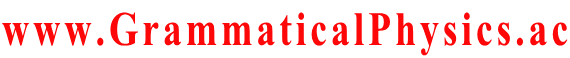Welcome SourceCodeOf_HumanGenome
www.GrammaticalPhysics.ac
Account
Access Detector
2 user(s) are online (1 user(s) are browsing Forum)

Members: 1
Guests: 1

SourceCodeOf_HumanGenome more...

# Theory of Quantum History Entangled in Time-like Direction

SourceCodeOf_HumanGenome > Examine Probability Formula @ 2013/5/28 16:29
Φ[χ] = Σj exp[α∫dt φj(χ(t), t)],

[dar(f; a, a + 1/α)]pre Φ[χ]
= ∫dx f(x)* Φ[ξ(□, x)]
= ∫dx f(x)* Σj exp[α∫dt φj(ξ(t, x), t)]
= Σj ∫dx f(x)* exp[α∫aa+1/α dt φj(x, t)] exp[α∫t'＜a or t'≧a+1/α dt' φj(χ(t'), t')]

dar(f; a, a + 1/α) Φ[χ(a,a+1/α)]
= [dar(f; a, a + 1/α)]pre Φ[χ]
= Σj ∫dx f(x)* exp[α∫aa+1/α dt φj(x, t)] exp[α∫t'＜a or t'≧a+1/α dt' φj(χ(t'), t')]
= Σj ∫dx f(x)* exp[α∫aa+1/α dt φj(x, t)] exp[α∫-∞a dt' φj(a,a+1/α)(t'), t') + α∫a dt' φj(a,a+1/α)(t'), t'+1/α)]
≒ Σj ∫dx f(x)* exp φj(x, a)
exp[ Σk＜0 φj(a,a+1/α)(a + k/α), a + k/α) + Σk≧0 φj(a,a+1/α)(a + k/α), a + (k + 1)/α)] ∵ α ≫ 1

dar(f; a, a + 1/α) Φ[χ]
≒ Σj ∫dx f(x)* exp φj(x, a) exp[ Σk＜0 φj(χ(a + k/α), a + k/α) + Σk≧0 φj(χ(a + k/α), a + (k + 1)/α)]

Let exp φ'j be a solution of the old Schrödinger equation for each j.
Let {exp φ'1(□, t), exp φ'2(□, t), ・・・} be an orthonormal basis of the state space of the old quantum mechanics for each t.
Let exp φj be a wave function given from exp φ'j by the twist remove normalization for each j.

∫Dχ dar(g; b, b + 1/α) Φ[χ]・{dar(f; a, a + 1/α) Φ[χ]}*
≒ Σj,j'k∫dχ(a+k/α)]
∫dx' g(x')* exp φj'(x', b) exp[ Σk'＜0 φj'(χ(b + k'/α), b + k'/α) + Σk'≧0 φj'(χ(b + k'/α), b + (k' + 1)/α)]
{∫dx f(x)* exp φj(x, a) exp[ Σk＜0 φj(χ(a + k/α), a + k/α) + Σk≧0 φj(χ(a + k/α), a + (k + 1)/α)]}*
= Σj,j' ∫dx' g(x')* exp φj'(x', b) ∫dx f(x) [exp φj(x, a)]*
Πk＜0 ∫dχ(a+k/α) [exp φj(χ(a + k/α), a + k/α)]* [exp φj(χ(a + k/α), a + k/α)]
Π0≦k＜α(b-a) ∫dχ(a+k/α) [exp φj(χ(a + k/α), a + (k + 1)/α)]* [exp φj(χ(a + k/α), a + k/α)]
Πk≧α(b-a) ∫dχ(a+k/α) [exp φj(χ(a + k/α), a + k/α)]* [exp φj(χ(a + k/α), a + k/α)]
= Σj,j' ∫dx' g(x')* exp φj'(x', b) ∫dx f(x) [exp φj(x, a)]*
(δj,j') Π0≦k＜α(b-a) ∫dχ(a+k/α) [exp φj(χ(a + k/α), a + (k + 1)/α)]* [exp φj(χ(a + k/α), a + k/α)]
= Σj ＜g|j,b＞＜j,a|f＞ Π0≦k＜α(b-a) ＜j,a+(k+1)/α|j,a+k/α＞
≒ Σj ＜g|j,b＞＜j,a|f＞ ∵ ※
= Σj ＜g|U(b,a)|j,a＞＜j,a|f＞
= ＜g|U(b,a)|f＞
if α(b - a) ∈ N.

---※---
Π0≦k＜α(b-a) ∫dχ(a+k/α) [exp φj(χ(a + k/α), a + (k + 1)/α)]* [exp φj(χ(a + k/α), a + k/α)]
= Π0≦k＜α(b-a) ＜j,a+(k+1)/α|j,a+k/α＞
≒ Π0≦k＜α(b-a) ＜j,a+k/α|j,a+k/α＞ ∵the twist remove normalization
= ＜j,a|j,a＞α(b-a)
= 1
---

However, notice that the new grammar version of the Schrödinger equation has no solution such that Φ[χ] = Σj exp[α∫dt φj(χ(t), t)].

A few approximations caused by the finiteness of α shift the result from the one in the old quantum mechanics.
Such an approximation is not needed if α is infinity.
I don't think that it is a prediction of the new theory distinct from the old quantum mechanics.
I think that it should be understood as the fact that a quantum history and a measurement are less related to each other than in the old quantum mechanics.

A prediction of the new theory distinct from the old quantum mechanics will be caused by the entanglement of a quantum history in a time-like direction.

---
Last edited at 2013/06/04/15:35JST
SourceCodeOf_HumanGenome > Twist Remove Normalization @ 2013/5/27 11:23
I suppose that a general definition of the twist remove normalization for a functional Φ is given by the condition:
∫Dχ Φ[χ]* Φ[χ] = 1,
(d/dε) ∫Dχ Φ[χ]* Φ[χ(□ - ε)] |ε = 0 = 0,
where χ(□ - ε) is a function such that
[χ(□ - ε)](t) = χ(t - ε) for all t.

Possibly, a general definition of the twist remove normalization for a functional Φ is given by the condition:
∫Dχ Φ[χ]* Φ[χ] = 1,
limε → 0 ∫Dχ Φ[χ]* Φ[χ(□ - ε)] = 1
because the phase factor exp[-⊿t(i/h)＜j|H]|j＞] in Problems in Grammatical Physics > Quantum Field Theory on the Time-Axis > Quadratic Formula corresponds not to (d/dε) ∫Dχ Φ[χ]* Φ[χ(□ - ε)] |ε = 0 but to limε → 0 ∫Dχ Φ[χ]* Φ[χ(□ - ε)].

Possibly, the twist remove normalization may not be necessary for Φ in the case that we use exp∫dt instead of Πt.

When
Φ[χ] = Σj Πk φj(χ(k/α), k/α),
change of the phase factor of φj
φ'j(χ(k/α), k/α) = exp[iFj(k/α)]φj(χ(k/α), k/α)
changes only the whole phase factor by exp[iΣkFj(k/α)].
Different paterns of changing phase factors without changing the whole phase factor can not be distinguished.
This concerns the energy ambiguity problem.

---
Last edited at 2013/05/28/17:08JST
SourceCodeOf_HumanGenome > Line Integral in Function Space @ 2013/5/26 16:32
ξ(□, x) represents a function which is a point in the function space for each x.

So, ξ is a parametric representation of a line in the function space, and x is a parameter of it.

By thinking of t as an index of a coordinate in the function space, η(x) = √{α∫dt [∂ξ(t,x)/∂x]2}
is understood as ds/dx where ds is the length of a line element.

η(x) is chosen to be 1 in the definition of the probability formula.

[dar(f; a, a + 1/α)]pre Φ[χ]
= ∫dx η(x) f(x)* Φ[ξ(□,x)]
= ∫dx f(x)* Φ[ξ(□,x)]

---
Last edited at 2013/05/31/17:34JST
SourceCodeOf_HumanGenome > Probability Formula in New Grammar @ 2013/5/18 13:32
Let Φ be a normalized functional representing a quantum history.
Let the twist remove normalization be used there.

Then the function, representing a transition amplitude of the new grammatical quantum mechanics, corresponding to the Green's function of the old quantum mechanics, is given by the following formula.

Γ(g, t2; f, t1) = ∫Dχ dar(g; t2, t2 + 1/α)Φ[χ]・{dar(f; t1, t1 + 1/α)Φ[χ]}*.
This function corresponds to ＜g, t2| f, t1＞ of the old quantum mechanics.

dar(f; a, b) is the stacked Daruma game operator defined as follows.

[dar(f; a, b)]pre Φ[χ] = ∫dx f(x)* Φ[ξ(□,x)]
where ξ is a function such that
ξ(t,x) = χ(t)　(t ＜ a or t ≧ b),
ξ(t,x) = x　(a ≦ t < b),
ξ(t,x) must be smoothened at t = a and t =b.

[dar(f; a, b)] Φ[χ(a,b)] = [dar(f; a, b)]pre Φ[χ]
where
χ(a,b)(t) = χ(t)　(t ＜ a),
χ(a,b)(t) = χ(t + (b - a))　(t ≧ a).

The twist remove normalization for a quantum history written in the form:
Φ[χ] = Σj exp[α∫dt φj(χ(t),t)]
is defined by the condition:
(∂/∂ε)∫dx [exp φj(x,t)]* exp φj(x,t + ε) |ε = 0 = 0.
This condition is satisfied with the function:
φj(x,t) = φ'j(x,t) + (i/h)t∫dx' [exp φ'j(x',t)]* H exp φ'j(x',t)
where exp φ'j is a solution of the old Schrödinger equation and H is the Hamiltonian of it.
That is to say,
φj(x,t) = φ'j(x,t) + (i/h)t＜j|H|j＞.

However to define the twist remove normalization generally is a remaining problem.
---
Last edited at 2013/06/04/15:58JST
SourceCodeOf_HumanGenome > Cartoon of the Equation @ 2013/4/3 14:39
I showed a problem of classical mechanics and its solutions as a cartoon of the contents of the reduced form of the new equation at JPS 2013 Spring Meeting.

It originated from the idea on 2011/08/02.

When time is descretized and V = 0, the reduced form of the new grammar version of the Schrodinger equation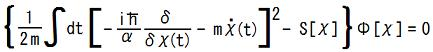is rewitten as follows.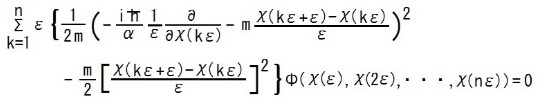---
Last edited at 2013/04/09/16:32JST
SourceCodeOf_HumanGenome > Similar problem in the old theories @ 2011/10/26 15:44
The equation for the function fne, k is rewritten as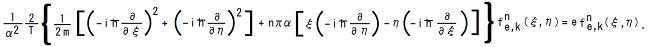This is the Schrodinger equation in the old quantum mechanics for a 2-dimensional system with Hamiltonian: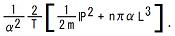The classical Hamiltonian of this system is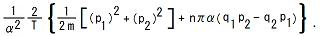So the classical equations of motion of this system are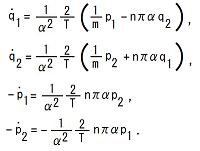To solve this equation is not difficult.
The solution is as follows.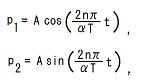and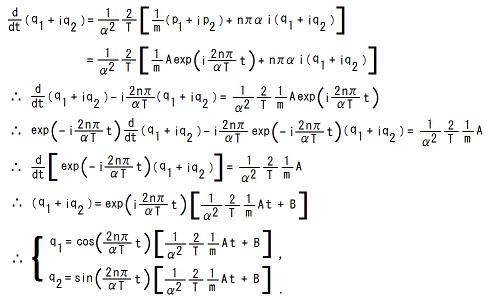Thus the first equation in this message seems not to be pathological.
---
Last edited at 2011/11/05/14:41JST
SourceCodeOf_HumanGenome > Solution by using circular time in the case V=0 @ 2011/10/12 10:38
If we choose functions f0k0, f1e1,k1, f2e2,k2,・・・ and coefficients c(k0; e1, k1; e2, k2; ・・・) as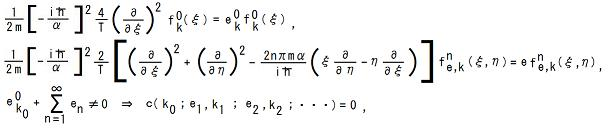then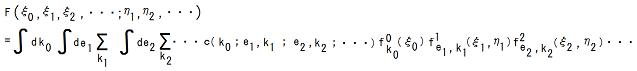is a solution of the new grammar version of Schrodinger equation in the case V=0.
The equation for the function f0k is easily solved as follows.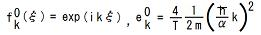where k is an arbitrary real number.
The equation for the function fne, k is solved as follows which was presented at JPS 2010 Autumn Meeting by Yuichi Uda.
By using Fourier expansion of the function fne, k: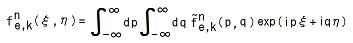the equation for the function fne, k is rewritten as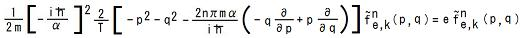To solve this equation, I introduced polar coordinates r and θ in pq-plane as p = r cosθ and q = r sinθ.
Then the equation for the function fne, k reduces to the following expression.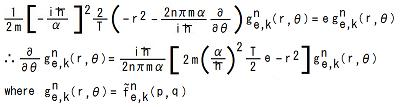This equation has the following solution.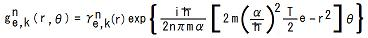Because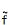must be continuous, g must be periodic in θ.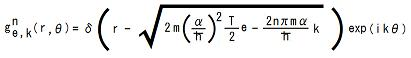where e is an arbitrary real number and k is an arbitrary integer which satisfies:---
Last edited at 2011/10/26/15:18JST
SourceCodeOf_HumanGenome > Circular Time Regularization @ 2011/8/24 10:54
The circular time was introduced by Yuichi Uda at JPS 2009 Autumn Meeting 13pSH-3 to solve the new grammar version of Schrödinger equation.

When we adopt the circular time, a functional Φ representing a quantum history can be expressed by a function of Fourier coefficients as follows.
Φ[χ] = F(a0[χ],a1[χ],a2[χ],・・・;b1[χ],b2[χ],・・・)
where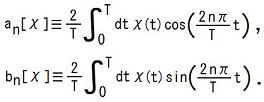Then the functional derivative with respect to χ(t) is written in terms of partial derivatives with respect to the Fourier coefficients.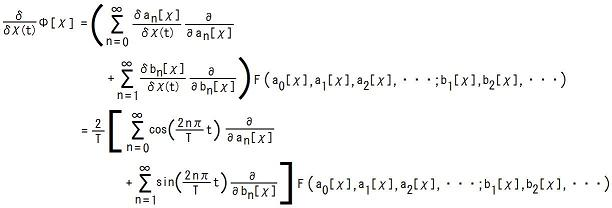By using orthogonality of trigonometric functions: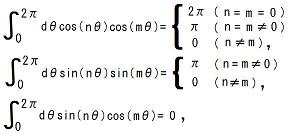it follows that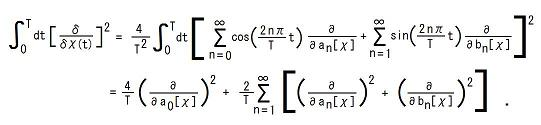On the other hand,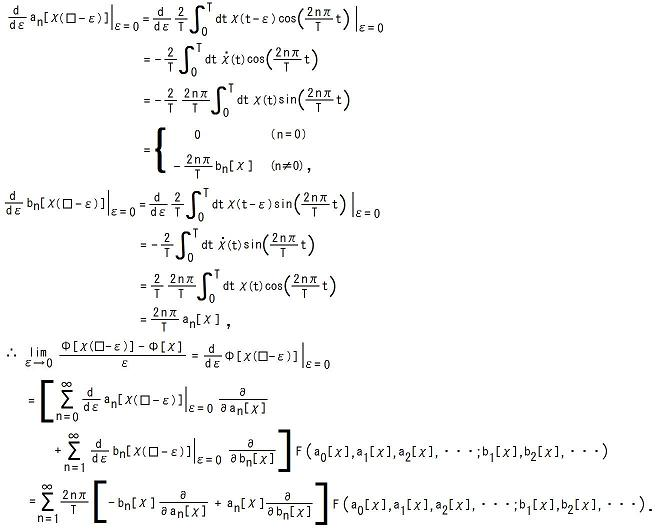Then the new grammar version of Schrödinger equation is rewritten as follows.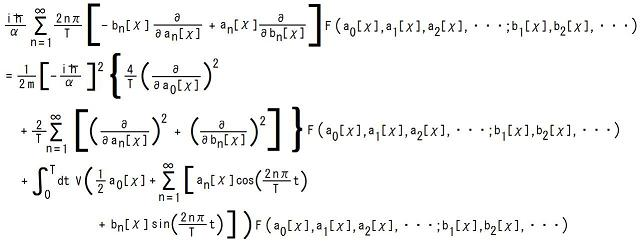Reducing this equation, it follows that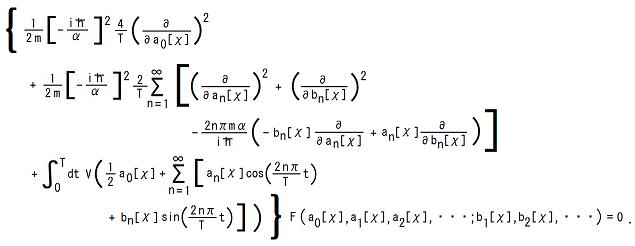That is,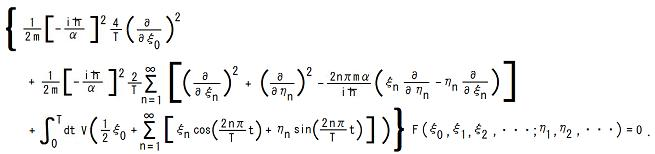---
Last edited at 2011/10/11/14:41JST
SourceCodeOf_HumanGenome > Unentangled Fundamental Solution @ 2011/8/22 15:32
Yuichi Uda found an unentangled fundamental solution on 2011/04/13.
This solution is written as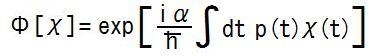for the new grammar version of Schrödinger equation: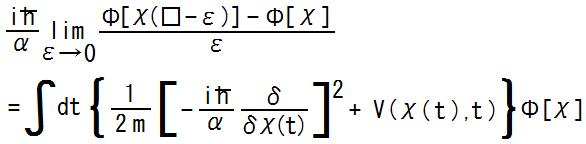when V(x;t) = -[1/(2m)][p(t)]2 - [dp(t)/dt]x.
---
Last edited at 2011/08/23/15:07JST

SourceCodeOf_HumanGenome > Meta Gauge Field @ 2011/8/21 10:32
The reduced form of the new grammar version of Schrödinger equation is analogous to the ordinary Schrödinger equation: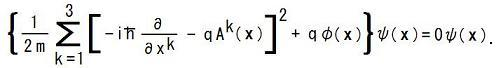This equation is for a stationary quantum state with energy eigenvalue zero.
mdχ(t)/dt is analogous to qAk(x) and -S[χ] is analogous to qφ(x).
This correspondence is fully understood by writing
qAt[χ] = mdχ(t)/dt, qφ[χ] = -S[χ].
---
Last edited at 2011/08/22/10:26JST

Category
Forum
Global

Notification method is private message [Change]

Site Search
Site Info
Webmasterswooder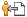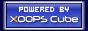Recommend Us